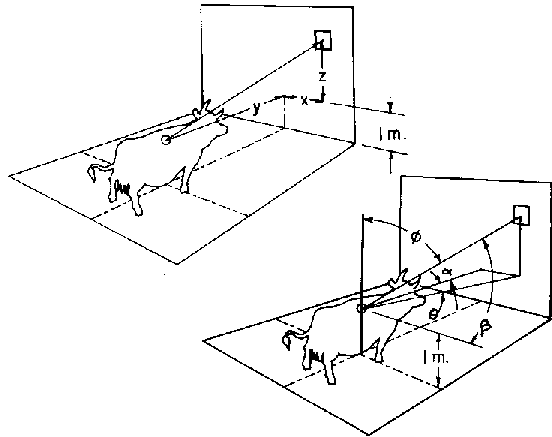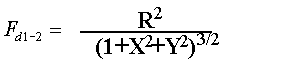>

# SECTION B Factors From Differential Elements to Finite Areas

## B-68: Differential planar element on ceiling, floor or any wall to cow. (x, y in meters)Definitions: R=r/1m; X=x/1m; Y=y/1m; R2=3.35+2.25cosb+0.74cosa+0.09cosf

Governing equation:NOTE: This is an approximate relation derived by fitting the equation for an equivalent sphere of radius R meters to measured values for a cow.
To download a copy of this equation in MS Word 97 Format, click HERE.